# Interest 1 Interest 2 Equated monthly installments Introduction

• Slides: 22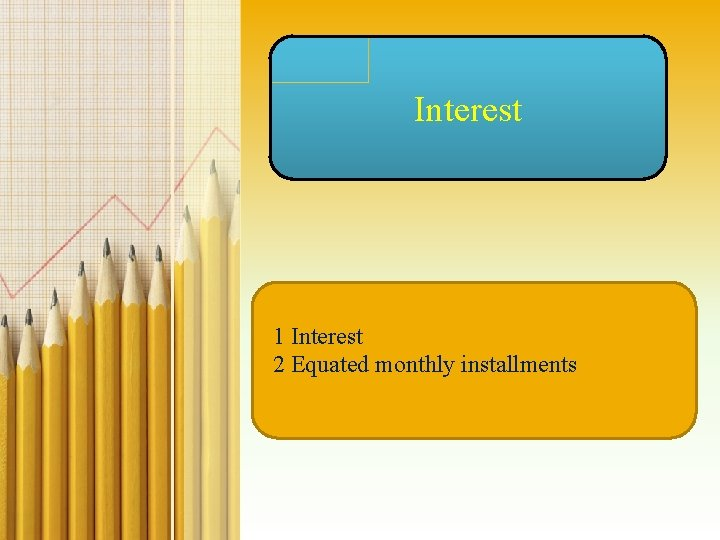Interest 1 Interest 2 Equated monthly installments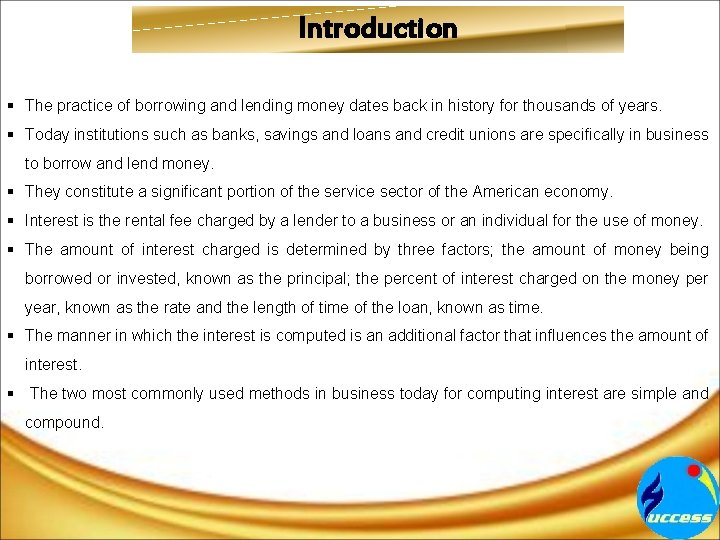Introduction § The practice of borrowing and lending money dates back in history for thousands of years. § Today institutions such as banks, savings and loans and credit unions are specifically in business to borrow and lend money. § They constitute a significant portion of the service sector of the American economy. § Interest is the rental fee charged by a lender to a business or an individual for the use of money. § The amount of interest charged is determined by three factors; the amount of money being borrowed or invested, known as the principal; the percent of interest charged on the money per year, known as the rate and the length of time of the loan, known as time. § The manner in which the interest is computed is an additional factor that influences the amount of interest. § The two most commonly used methods in business today for computing interest are simple and compound.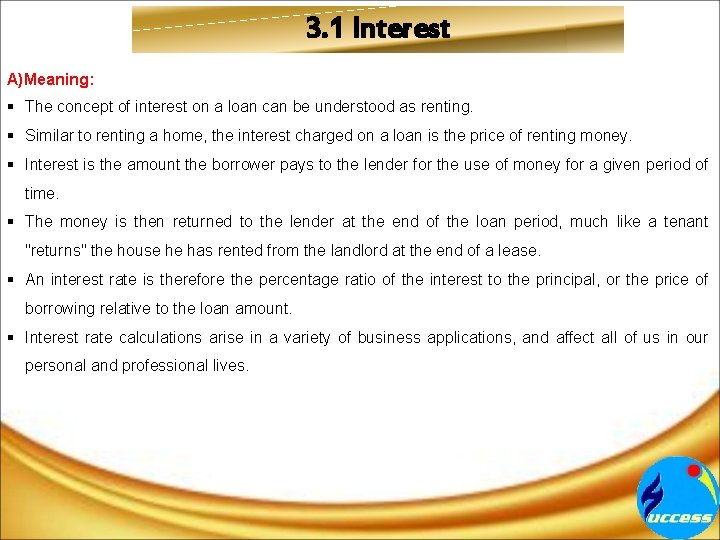3. 1 Interest A)Meaning: § The concept of interest on a loan can be understood as renting. § Similar to renting a home, the interest charged on a loan is the price of renting money. § Interest is the amount the borrower pays to the lender for the use of money for a given period of time. § The money is then returned to the lender at the end of the loan period, much like a tenant "returns" the house he has rented from the landlord at the end of a lease. § An interest rate is therefore the percentage ratio of the interest to the principal, or the price of borrowing relative to the loan amount. § Interest rate calculations arise in a variety of business applications, and affect all of us in our personal and professional lives.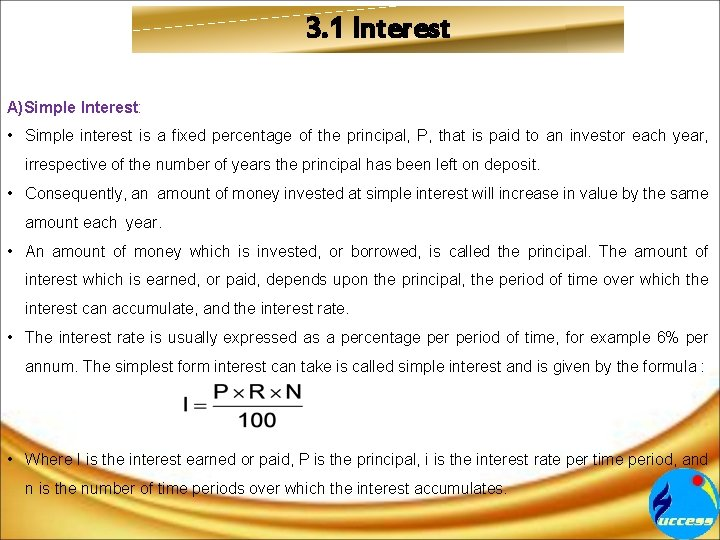3. 1 Interest A)Simple Interest: • Simple interest is a fixed percentage of the principal, P, that is paid to an investor each year, irrespective of the number of years the principal has been left on deposit. • Consequently, an amount of money invested at simple interest will increase in value by the same amount each year. • An amount of money which is invested, or borrowed, is called the principal. The amount of interest which is earned, or paid, depends upon the principal, the period of time over which the interest can accumulate, and the interest rate. • The interest rate is usually expressed as a percentage period of time, for example 6% per annum. The simplest form interest can take is called simple interest and is given by the formula : • Where I is the interest earned or paid, P is the principal, i is the interest rate per time period, and n is the number of time periods over which the interest accumulates.3. 1 Interest Example : 1) If an amount of Rs. 4000 is invested in a savings account at an interest rate of 8% per year, calculate the (simple) interest paid over a three year period. Solution : Here P = Rs. 4000, i = 8% = 0. 08, and n = 3. So I = Pin = (4000)(0. 8)(3) = Rs. 960 This is equivalent to earning interest of Rs. 320 in each of the three years. B)Compound interest : It refers to interest applied to the principal, in addition to unpaid interest added to the principal. These factors lead to the formula 1) FV =Future value of the deposit P=Principal or amount of money deposited r = annunal interest rate (in decimal form) n =number of times compounded per year t = time in years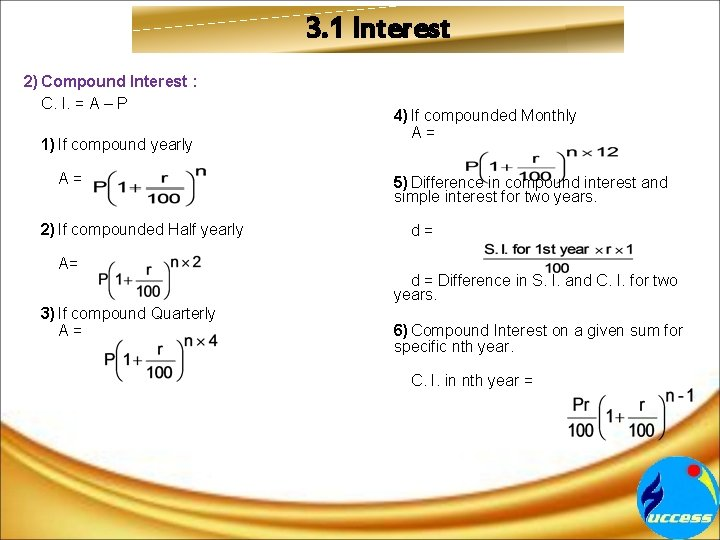3. 1 Interest 2) Compound Interest : C. I. = A – P 4) If compounded Monthly A= 1) If compound yearly A= 5) Difference in compound interest and simple interest for two years. 2) If compounded Half yearly d= A= d = Difference in S. I. and C. I. for two years. 3) If compound Quarterly A= 6) Compound Interest on a given sum for specific nth year. C. I. in nth year =3. 1 Interest 1)Types of Compound Interest : a)Nominal Rate of Interest : Nominal interest rate refers to the rate of interest prior to taking inflation into account. Nominal Interest Rate = Real Interest Rate + Inflation Premium + Risk Premium. Calculating Nominal Interest Rate : Nominal interest rate for a period with effective interest rates for it’s sub periods can be calculated as i= (1+i ) -1 e (1) n where i = nominal interest rate for the period i = effective interest rate for the subperiod e n = number of subperiod Example - Nominal interest rate with effective monthly interest rates Nominal interest rate (per year) with monthly effective rates of 1% can be calculated as i = (1+0. 010) -1 n 12 = 0. 127 = 12. 7%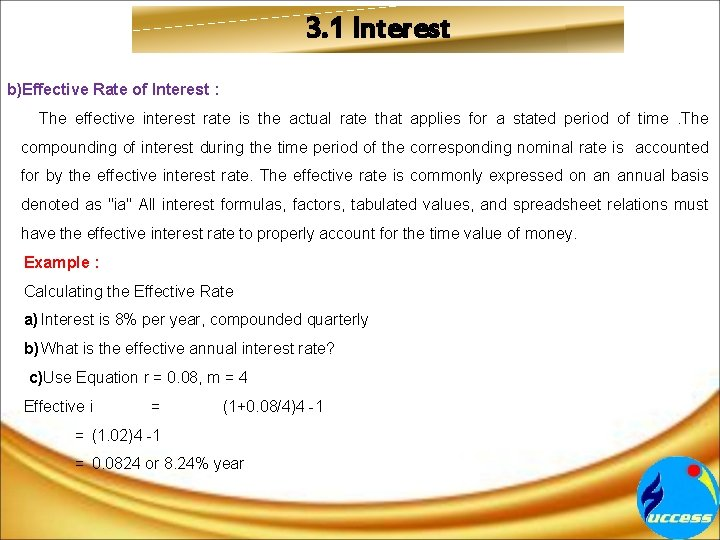3. 1 Interest b)Effective Rate of Interest : The effective interest rate is the actual rate that applies for a stated period of time. The compounding of interest during the time period of the corresponding nominal rate is accounted for by the effective interest rate. The effective rate is commonly expressed on an annual basis denoted as "ia" All interest formulas, factors, tabulated values, and spreadsheet relations must have the effective interest rate to properly account for the time value of money. Example : Calculating the Effective Rate a) Interest is 8% per year, compounded quarterly b) What is the effective annual interest rate? c)Use Equation r = 0. 08, m = 4 Effective i = (1+0. 08/4)4 -1 = (1. 02)4 -1 = 0. 0824 or 8. 24% year3. 2 Equated Monthly Instalments (EMIs) A)Meaning: They are the monthly payouts that go towards paying a loan. They consist of both the principal and interest that needs to be repaid. Equated monthly installments are used to pay off both interest and principal each month, so that over a specified number of years, the loan is paid off in full. It further explains that, with most common types of loans, such as real estate mortgages, the borrower makes fixed periodic payments to the lender over the course of several years with the goal of retiring the loan. EMIs differ from variable payment plans, in which the borrower is able to pay higher payment amounts at his or her discretion. In EMI plans, borrowers are usually only allowed one fixed payment amount each month. 1) Formula to Calculate EMI : The formula for calculation of EMI given the loan, term and interest rate is: E= P x r x (1 + r)n / ((1+r)n -1) Here p = principal amount r = interest rate per month (ex: if interest rate per annum is 10% then 10/(12*100)) n = tenure in months / no. of instalments in the tenure,3. 2 Equated Monthly Instalments (EMIs) A)Interest Rates of Equated Monthly Installments (EMIs) : An interest rate of Equated Monthly Installments (EMIs) is the rate at which interest is paid by borrowers for the use of money that they borrow from a lender. Specifically, the interest rate (I/m) is a percent of principal (P) paid a certain amount of times (m) period (usually quoted per annum). 1)Flat Interest Rate : Flat Rate EMI's remain untouched through the entire repayment period. Flat interest rate is an interest rate calculated on the full original loan amount for the whole term without taking into consideration that periodic payments reduce the amount loaned. In other words, Flat Rate of Interest basically means that interest is charged on the full. 2)Reducing / Diminishing Interest Rate : Reducing balance is what you should look for as the calculation under this method is considered more favourable. The banks subtract the principal already paid through earlier EMI's and charge interest on the outstanding amount of principal. In Reducing / Diminishing Balance Rate method, interest is calculated every month on the outstanding loan balance. .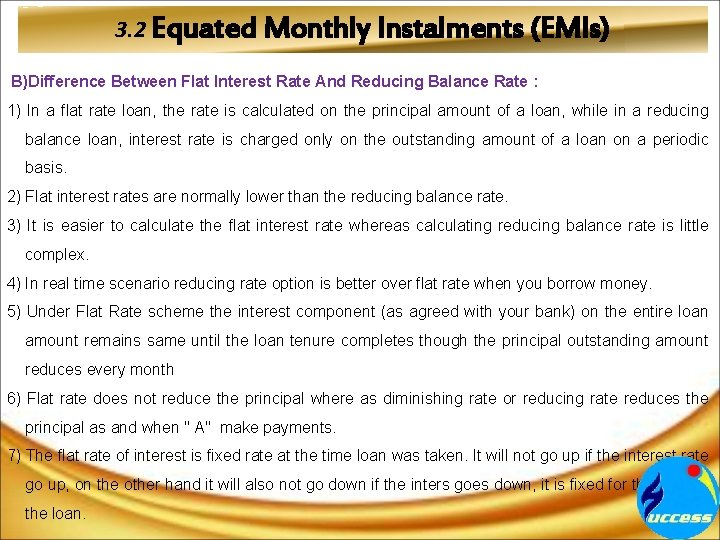3. 2 Equated Monthly Instalments (EMIs) B)Difference Between Flat Interest Rate And Reducing Balance Rate : 1) In a flat rate loan, the rate is calculated on the principal amount of a loan, while in a reducing balance loan, interest rate is charged only on the outstanding amount of a loan on a periodic basis. 2) Flat interest rates are normally lower than the reducing balance rate. 3) It is easier to calculate the flat interest rate whereas calculating reducing balance rate is little complex. 4) In real time scenario reducing rate option is better over flat rate when you borrow money. 5) Under Flat Rate scheme the interest component (as agreed with your bank) on the entire loan amount remains same until the loan tenure completes though the principal outstanding amount reduces every month 6) Flat rate does not reduce the principal where as diminishing rate or reducing rate reduces the principal as and when " A" make payments. 7) The flat rate of interest is fixed rate at the time loan was taken. It will not go up if the interest rate go up, on the other hand it will also not go down if the inters goes down, it is fixed for the term of the loan.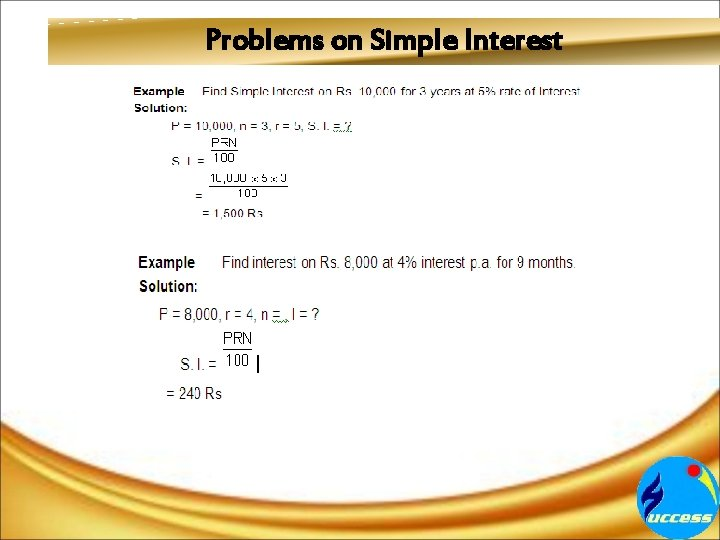Problems on Simple InterestProblems on Simple Interest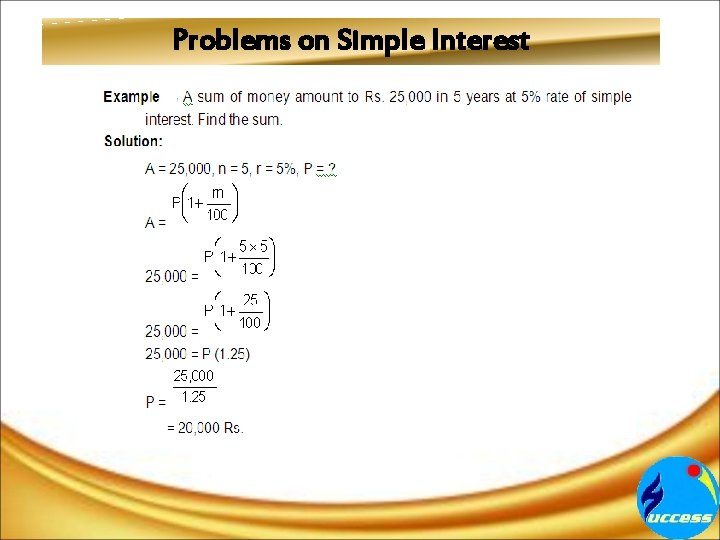Problems on Simple Interest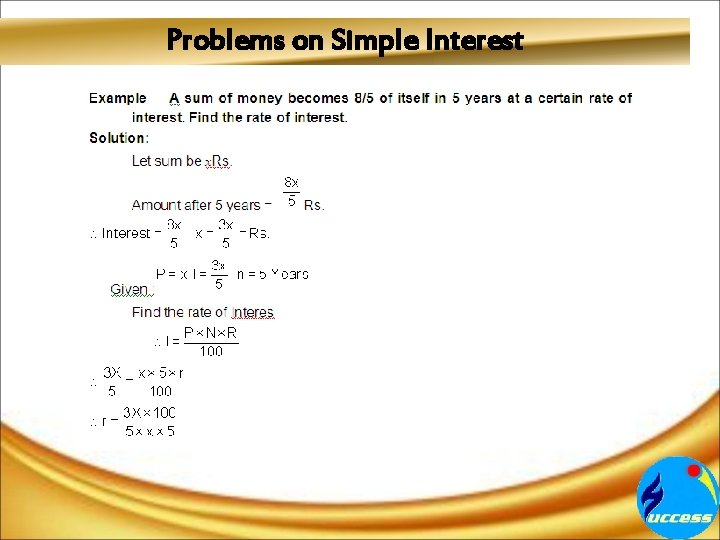Problems on Simple InterestProblems on Simple Interest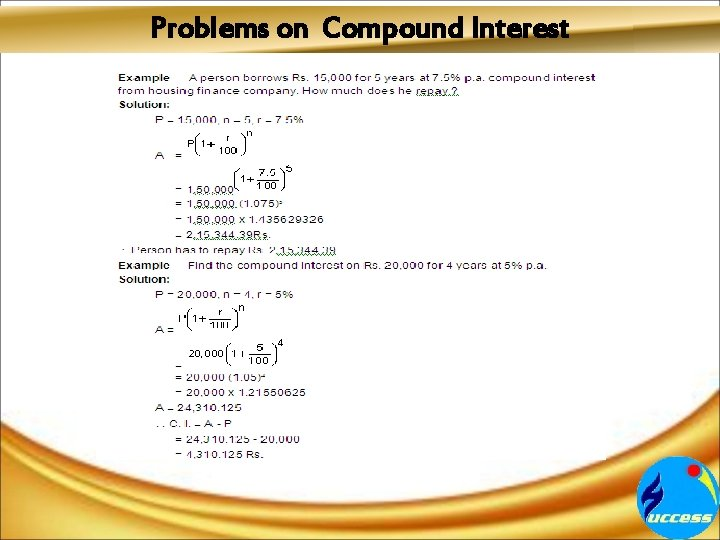Problems on Compound Interest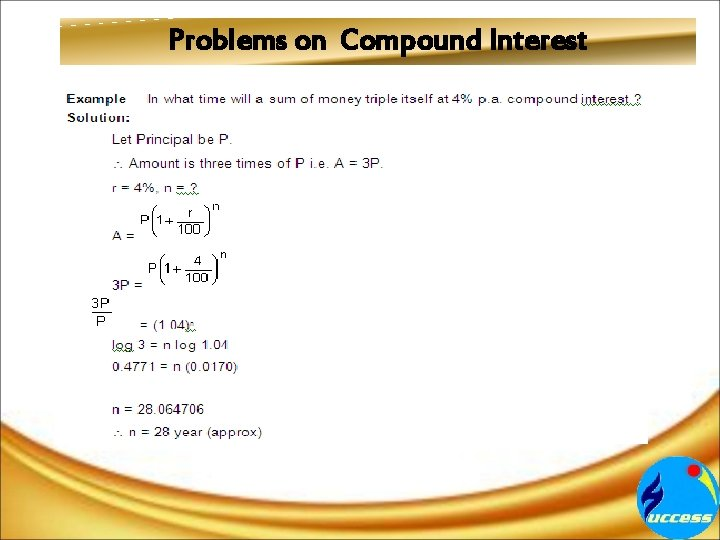Problems on Compound InterestProblems on Compound Interest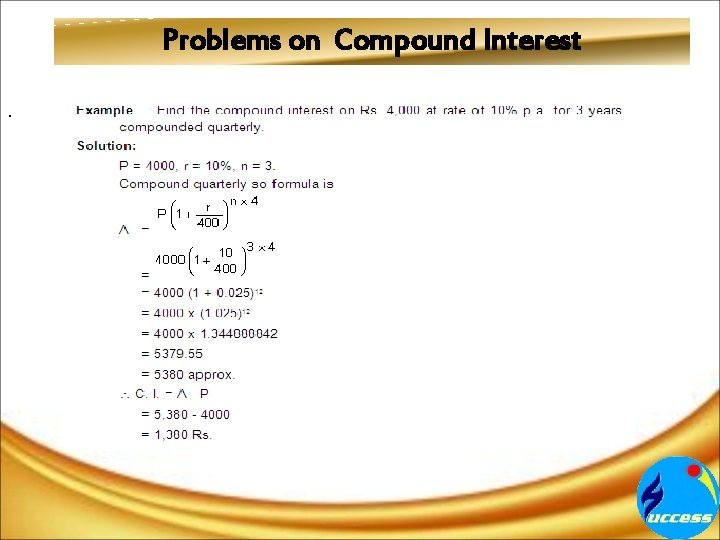Problems on Compound Interest.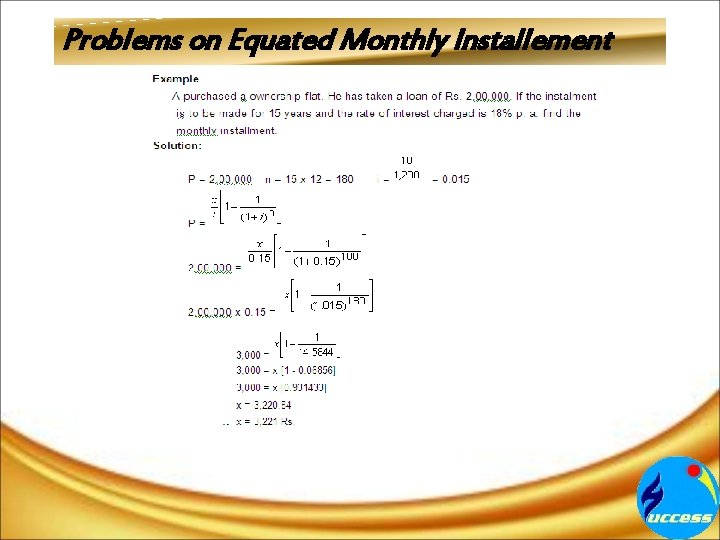Problems on Equated Monthly Installement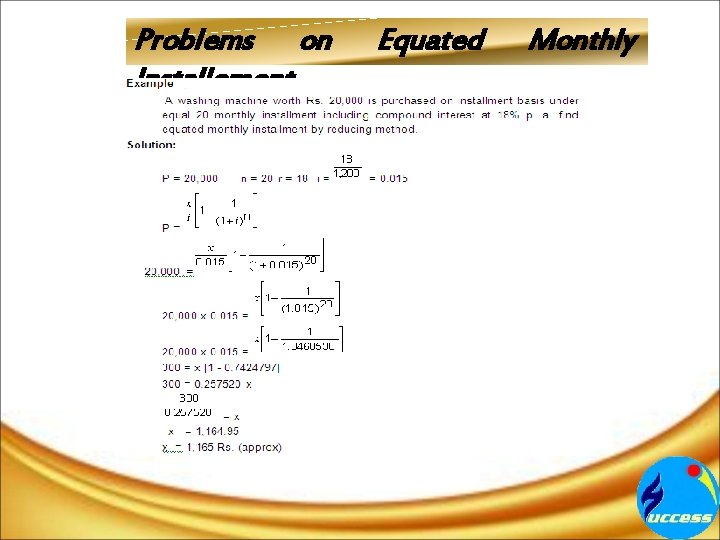Problems on Installement Equated Monthly